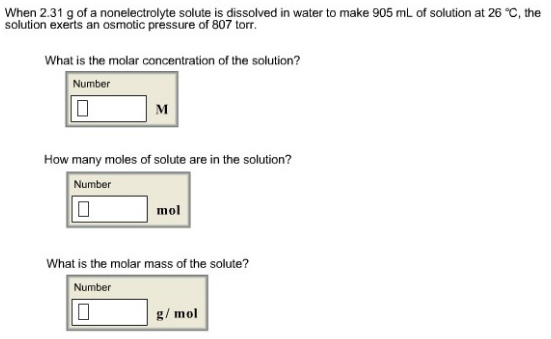# Problem: When 2.31 g of a nonelectrolyte solute is dissolved in water to make 905 mL of solution at 26°C, the solution exerts an osmotic pressure of 807 torr. What is the molar concentration of the solution? How many moles of solute are in the solution?

###### FREE Expert Solution
92% (415 ratings)###### Problem Details

When 2.31 g of a nonelectrolyte solute is dissolved in water to make 905 mL of solution at 26°C, the solution exerts an osmotic pressure of 807 torr.

What is the molar concentration of the solution?

How many moles of solute are in the solution?